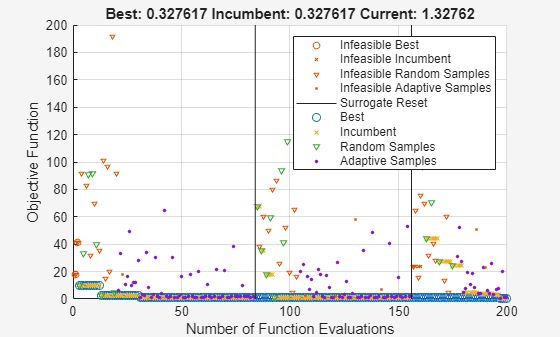Interpret surrogateoptplot

The surrogateoptplot plot function provides a good deal of information about the surrogate optimization steps.

Minimize Bounded Function

For example, consider the plot of the steps surrogateopt takes on the built-in test function rastriginsfcn. This function has a global minimum value of 0 at the point [0,0].

Create a surface plot of rastriginsfcn.

ezsurf(@(x,y)rastriginsfcn([x(:),y(:)]));Plot Minimization Process

By giving asymmetric bounds, you encourage surrogateopt to search away from the global minimum. Set asymmetric bounds of [-3,-3] and [9,10]. Set options to use the surrogateoptplot plot function, and then call surrogateopt.

lb = [-3,-3];
ub = [9,10];
options = optimoptions('surrogateopt','PlotFcn','surrogateoptplot');
rng default
[x,fval] = surrogateopt(@rastriginsfcn,lb,ub,options);Surrogateopt stopped because it exceeded the function evaluation limit set by
'options.MaxFunctionEvaluations'.

Interpret Plot

Begin interpreting the plot from its left side. For details of the algorithm steps, see Surrogate Optimization Algorithm.

• The first points are black triangles, indicating quasirandom samples of the function within the problem bounds. These points come from the Construct Surrogate phase.

• Next are black dots indicating the adaptive points, the points created in the Search for Minimum phase.

• The thick green line represents the best (lowest) objective function value found. Shortly after evaluation number 30, surrogateopt is stuck in a local minimum with an objective function value near 5. Zoom in to see this behavior more clearly.

xlim([20 100])
ylim([0 10])• Near evaluation number 120, a vertical line indicates a surrogate reset. At this point, the algorithm returns to the Construct Surrogate phase.

• The dark blue x points represent the incumbent, which is the best point found since the previous surrogate reset.

• Near evaluation number 160, the incumbent improves on the previous best point by attaining a value of about 4. After this evaluation number, the best point slowly drops in value. Zoom in to see this behavior more clearly.

xlim([140 200])
ylim([0 6])• The optimization halts at evaluation number 200 because it is the default function evaluation limit for a 2-D problem.

Problem with Nonlinear Constraints

The surrogateoptplot display changes when you have nonlinear constraints. Impose the constraint that x(1) is integer-valued, and the nonlinear constraint that ${x}_{2}\ge {x}_{1}^{2}-2$. For the function that implements this constraint, see rasfcn at the end of this example.

fun = @rasfcn;

Set integer constraints by setting intcon = 1, and run the minimization.

intcon = 1;
[x,fval] = surrogateopt(fun,lb,ub,intcon,options);Surrogateopt stopped because it exceeded the function evaluation limit set by
'options.MaxFunctionEvaluations'.

The plot now shows red markers where surrogateopt evaluates an infeasible point. The final point is close to the true minimum point of [0,0].

disp(x)
1.0e-03 *

0   -0.3913

The integer constraint likely helps surrogateopt find the true minimum, by reducing the search space.

function F = rasfcn(x)
F.Fval = rastriginsfcn(x);
F.Ineq = x(1)^2 - 2 - x(2);
end